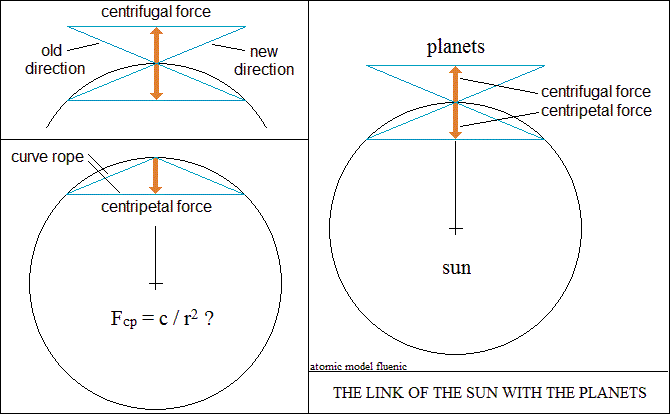Interactions in vector space.
The centripetal and centrifugal forces are generated "in the mirror"
by the same phenomenon, by the direction property of the vectors.
The maximum attraction orients the vectors in the same direction, collinear.
Centripetal force
In purely vector closed circuits, called electrical and magnetic,
this property transforms the curve arc in a straight line into a string.
In this way, the circuit compresses to the center with the speed of light.
The force of attraction between the vectors are now converted into the centripetal force.
Centrifugal force
Centrifugal force is the reaction of vector space to the movement of the body (open circuit).
Linear motion orients space vectors in the same direction, a phenomenon called inertia.
Changing of the direction of motion also changes the direction of the orientation of spatial vectors.
The two vector directions tangent to the curve form two "in mirror" angles.
As with closed circuits, the angles become equal strings and forces with opposite directions.
The outer rope represents the centrifugal force.
The inner rope represents the centripetal force, which curves the movement of the body.
The interpretation shows the close connection between centripetal force and centrifugal force.
From experiments, the centrifugal force depends on the velocity
of the body and the angle of the two tangents, respectively on the radius of curvature.
So the centrifugal force is proportional to the velocity and inversely proportional to the radius Fcf = v / r
In the case of centripetal force, the speed of vector attraction being
the speed of light, the law of universal attraction becomes: Fcp = c / r2<>